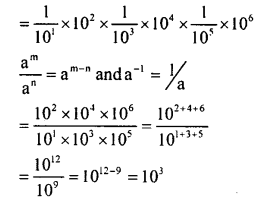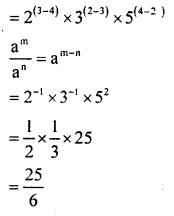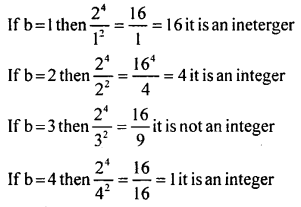# KSEEB Solutions for Class 8 Maths Chapter 10 Exponents Ex 10.3

Students can Download Maths Chapter 10 Exponents Ex 10.3 Questions and Answers, Notes Pdf, KSEEB Solutions for Class 8 Maths helps you to revise the complete Karnataka State Board Syllabus and score more marks in your examinations.

## Karnataka Board Class 8 Maths Chapter 10 Exponents Ex 10.3

Question 1.
Simplify:
(i) 10-1 x 102 x 10-3 x 104 x 105 x 106
(ii) $$\frac{2^{3} \times 3^{2} \times 5^{4}}{3^{3} \times 5^{2} \times 2^{4}}$$
(i) 10-1 x 102 x 10-3 x 104 x 105 x 106(ii) $$\frac{2^{3} \times 3^{2} \times 5^{4}}{3^{3} \times 5^{2} \times 2^{4}}$$Question 2.
Which is larger (34 × 23) or (25 × 32)
34 × 23 = 81 × 8 = 648
25 × 32 = 32 × 9 = 288
648 > 288
34 × 2> 25 × 32

Question 3.
Suppose ‘m’ and ‘n’ are distinct integers  $$\frac{3^{m} \times 2^{n}}{2^{m} \times 3^{n}}$$  can be an integer? Give reasons.$\frac{3^{m} \times 2^{n}}{2^{m} \times 3^{n}} = \frac{3^{m-n}}{2^{m-n}}$
If ‘m’ and ‘n’ are two distinct integers.
Then 3(m-n) is always on an odd number and 2(m-n) is always an even number.
If an odd number is divided, by an even number the quotient is not an integer.
Therefore it is’ not an integer.

Question 4.
Suppose b is a positive integer such that is also an integer. What are the possible values of b.